JEE  >  JEE Advanced Test- 11

# JEE Advanced Test- 11 - JEE

Test Description

## 72 Questions MCQ Test JEE Main & Advanced Mock Test Series - JEE Advanced Test- 11

JEE Advanced Test- 11 for JEE 2023 is part of JEE Main & Advanced Mock Test Series preparation. The JEE Advanced Test- 11 questions and answers have been prepared according to the JEE exam syllabus.The JEE Advanced Test- 11 MCQs are made for JEE 2023 Exam. Find important definitions, questions, notes, meanings, examples, exercises, MCQs and online tests for JEE Advanced Test- 11 below.
 1 Crore+ students have signed up on EduRev. Have you?
JEE Advanced Test- 11 - Question 1

### A piston can freely move inside a horizontal cylinder closed from both ends. Initially, the piston separates the inside space of the cylinder into two equal parts each of  volume V0 , in which an ideal gas is contained under the same pressure p0 and at the same temperature. What work has to be performed  in order to increase isothermally the volume of one part of gas 2 times compared to that of the other by slowly moving the piston ?

Detailed Solution for JEE Advanced Test- 11 - Question 1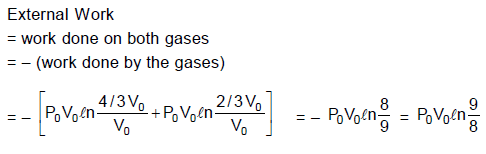JEE Advanced Test- 11 - Question 2

### A uniform pressure P is exerted by an external agent on all sides of a solid cube at temperature t ºC. By what amount should the temperature of the cube be raised in order to bring its volume back to its original volume before the pressure was applied if the bulk modulus is B and co-efficient of volumetric expansion is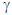?

Detailed Solution for JEE Advanced Test- 11 - Question 2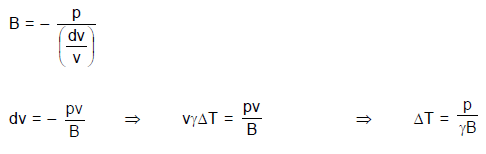JEE Advanced Test- 11 - Question 3

### Two rods of same dimensions, but made of different materials are joined end to end with their free ends being maintained at 100ºC and 0ºC respectively. The temperature of the junction is 70ºC. Then the temperature of the junction if the rods are interchanged will be equal to :

Detailed Solution for JEE Advanced Test- 11 - Question 3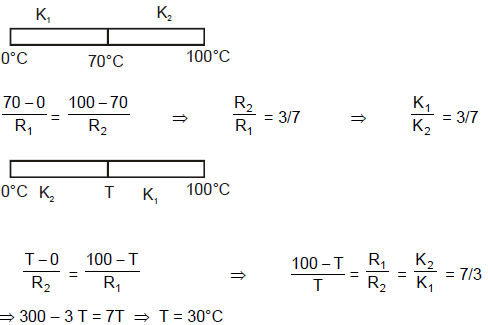JEE Advanced Test- 11 - Question 4

Assume a sample of an ideal gas in a vessel. Where velocity of molecules are between 2 m/sec to 5 m/sec and velocity of molecules (v) and number of molecules (n) are related as n = 7v – v2 – 10. The most probable velocity in sample is. Where v is measured in m/sec.

Detailed Solution for JEE Advanced Test- 11 - Question 4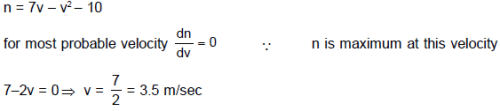JEE Advanced Test- 11 - Question 5

If specific heat capacity of a substance in solid and liquid state is proportional to temperature of the substance, then if heat is supplied to the solid initially at – 20°C (having melting point 0°C) at constant rate. Then the temperature dependence of substance with time will be best represented by :

Detailed Solution for JEE Advanced Test- 11 - Question 5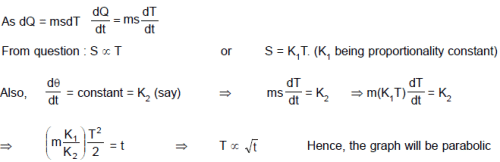JEE Advanced Test- 11 - Question 6

In the figure shown the pressure of the gas in state B is: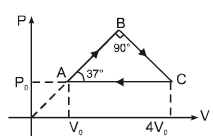Detailed Solution for JEE Advanced Test- 11 - Question 6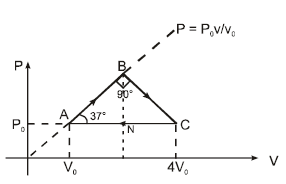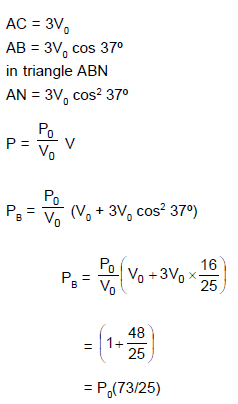JEE Advanced Test- 11 - Question 7

A hot black body emits the energy at the rate of 16 J m–2 s–1 and its most intense radiation corresponds to 20,000 Å. When the temperature of this body is further increased and its most intense radiation corresponds to 10,000 Å, then the energy radiated in Jm–2 s–1 will be :

Detailed Solution for JEE Advanced Test- 11 - Question 7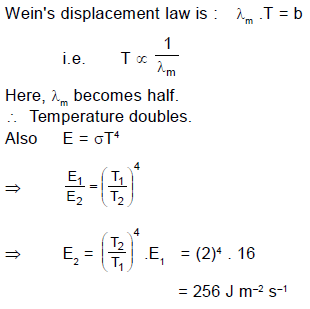JEE Advanced Test- 11 - Question 8

Two identical rectangular rods of metal are welded end to end in series between temperature 0°C and 100°C and 10 J of heat is conducted (in steady state process) through the rod in 2.00 min. If 5 such rods are taken and joined as shown in figure maintaining the same temperature difference between A and B,  then the time in which 20 J heat will flow through the rods  is :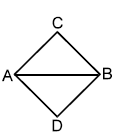Detailed Solution for JEE Advanced Test- 11 - Question 8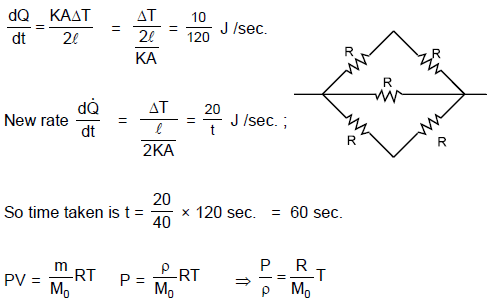*Multiple options can be correct
JEE Advanced Test- 11 - Question 9

An ideal gas undergoes a cyclic process abcda which is shown by pressure- density curve.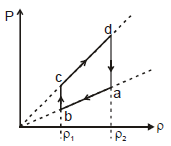Detailed Solution for JEE Advanced Test- 11 - Question 9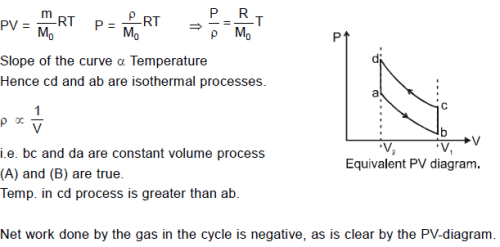*Multiple options can be correct
JEE Advanced Test- 11 - Question 10

The emissive power of a black body at T = 300 K is 100 Watt/m2. Consider a body B of area A = 10 m2 coefficient of reflectivity r = 0.3 and coefficient of transmission t = 0.5 and at temperature 300 K. Then which of the following is correct :

Detailed Solution for JEE Advanced Test- 11 - Question 10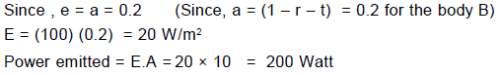*Multiple options can be correct
JEE Advanced Test- 11 - Question 11

The ends of a rod of uniform thermal conductivity are maintained at different (constant) temperatures. After the steady state is achieved :

Detailed Solution for JEE Advanced Test- 11 - Question 11

Heat obviously flows from higher temperature to lower temperature in steady state. ⇒ A is true.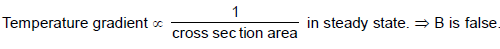Thermal current through each cross section area is same. ⇒ C is true.
Temperature decreases along the length of the rod from higher temperature end to lower temperature end. ⇒ D
is false.

*Multiple options can be correct
JEE Advanced Test- 11 - Question 12

Number of collisions of molecules of a gas on the wall of a container per m2 will :

Detailed Solution for JEE Advanced Test- 11 - Question 12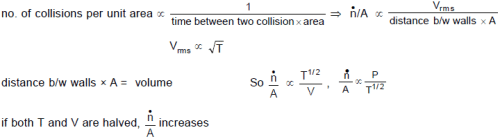JEE Advanced Test- 11 - Question 13

Statement -1 : It is possible for both the pressure and volume of a monoatomic ideal gas of a given amount to change simultaneously without causing the internal energy of the gas to change.

Statement-2: The internal energy of an ideal gas of a given amount remains constant if temperature does not change. It is possible to have a process in which pressure and volume are changed such that temperature remains constant.

Detailed Solution for JEE Advanced Test- 11 - Question 13

An isothermal process is an example to show the possibility "for both the pressure and volume of a monoatomic ideal gas to change simultaneously without causing the internal energy of the gas to change".
Hence Statement-1 is True, Statement-2 is True; Statement-2 is a correct explanation for Statement-1.

JEE Advanced Test- 11 - Question 14

Statement-1 : Molar heat capacity of an ideal monoatomic gas at constant volume is a constant at all temperatures.

Statement-2 : As the temperature of an monoatomic ideal gas is increased, number of degrees of freedom of gas molecules remains constant.

Detailed Solution for JEE Advanced Test- 11 - Question 14

For monoatomic gas the degrees of freedom are only translational and hence do not depend on temperature. Therefore molar heat capacity of an ideal monoatomic gas at constant volume is a constant at all temperatures.

JEE Advanced Test- 11 - Question 15

Statement-1 : Burns sustained from steam at 100ºC are usually more serious than those sustained by boiling water (at 100ºC)

Statement-2 : To convert 1gm of water at 100ºC to 1 gm of steam at 100ºC, 540 calories of heat is to be supplied. Hence 1gm of steam at 100ºC has more heat content than 1 gm of water at 100ºC

Detailed Solution for JEE Advanced Test- 11 - Question 15

Heat energy stored in 1 g of steam at 100°C is more in comparison to 1 g of water at 100°C. Hence burns caused by steam are more severe.

JEE Advanced Test- 11 - Question 16

Statement-1 : If the absolute temperature of a gas is doubled, the final rms-velocity of the gas particles becomes √2 times the initial value.
Statement-2: The average translational kinetic energy of molecules in a gas is equal to both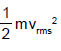and 3/2KT.( where vrms, k and T have usual meanings)

Detailed Solution for JEE Advanced Test- 11 - Question 16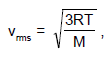if the absolute temperature T doubles, vrms must increase by √2 (m and k are constants).

JEE Advanced Test- 11 - Question 17

A 0.60 kg sample of water and a sample of ice are placed in two compartments A and B that are separated by a conducting wall, in a thermally insulated container. The rate of heat transfer from the water to the ice through the conducting wall is constant P, until thermal equilibrium is reached. The temperature T of the liquid water and the ice are given in graph as functions of time t. Temperature of the each compartment remain homogeneous during whole heat transfer process.
Given specific heat of ice = 2100 J/kg-K
Given specific heat of water = 4200 J/kg-K
Latent heat of fusion of ice = 3.3 × 105 J/kg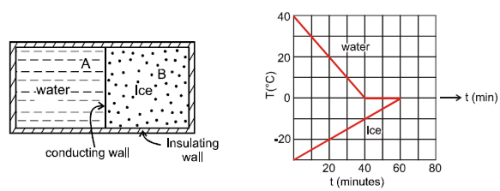Q. The value of rate P is

Detailed Solution for JEE Advanced Test- 11 - Question 17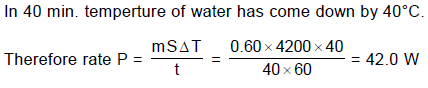JEE Advanced Test- 11 - Question 18

A 0.60 kg sample of water and a sample of ice are placed in two compartments A and B that are separated by a conducting wall, in a thermally insulated container. The rate of heat transfer from the water to the ice through the conducting wall is constant P, until thermal equilibrium is reached. The temperature T of the liquid water and the ice are given in graph as functions of time t. Temperature of the each compartment remain homogeneous during whole heat transfer process.
Given specific heat of ice = 2100 J/kg-K
Given specific heat of water = 4200 J/kg-K
Latent heat of fusion of ice = 3.3 × 105 J/kgQ. The initial mass of the ice in the container is equal to

Detailed Solution for JEE Advanced Test- 11 - Question 18

Sample of ice has been receiving heat at constant rate P from water. Its temperature has increased by 30°C in time 60 min.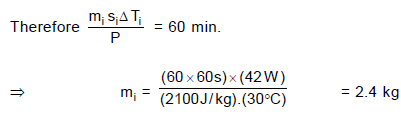JEE Advanced Test- 11 - Question 19

A 0.60 kg sample of water and a sample of ice are placed in two compartments A and B that are separated by a conducting wall, in a thermally insulated container. The rate of heat transfer from the water to the ice through the conducting wall is constant P, until thermal equilibrium is reached. The temperature T of the liquid water and the ice are given in graph as functions of time t. Temperature of the each compartment remain homogeneous during whole heat transfer process.
Given specific heat of ice = 2100 J/kg-K
Given specific heat of water = 4200 J/kg-K
Latent heat of fusion of ice = 3.3 × 105 J/kgQ. The mass of the ice formed due to conversion from the water till thermal equilibrium is reached, is equal to

Detailed Solution for JEE Advanced Test- 11 - Question 19

Thermal equilibrium reaches after 60 min. Ice conversion takes place for 20 min. During this time water at 0°C continues to give heat at rate P.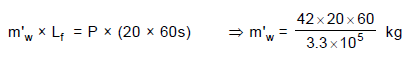= 0.15 kg

JEE Advanced Test- 11 - Question 20

A quantity of an ideal monoatomic gas consists of n moles initially at temperature T1. The pressure and volume both are then slowly doubled in such a manner so as to trace out a straight line on a P-V diagram.

Q. For this process, the ratio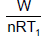is equal to (where W is work done by the gas) :

Detailed Solution for JEE Advanced Test- 11 - Question 20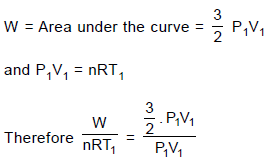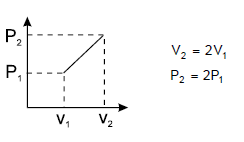JEE Advanced Test- 11 - Question 21

A quantity of an ideal monoatomic gas consists of n moles initially at temperature T1. The pressure and volume both are then slowly doubled in such a manner so as to trace out a straight line on a P-V diagram.

Q. For the same process, the ratio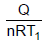is equal to (where Q is heat supplied to the gas) :

Detailed Solution for JEE Advanced Test- 11 - Question 21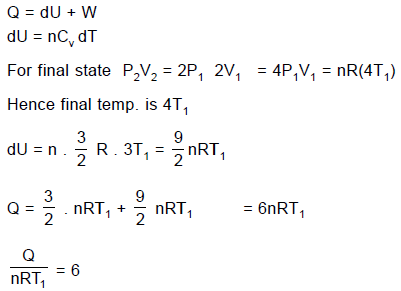JEE Advanced Test- 11 - Question 22

A quantity of an ideal monoatomic gas consists of n moles initially at temperature T1. The pressure and volume both are then slowly doubled in such a manner so as to trace out a straight line on a P-V diagram.

Q. If C is defined as the average molar specific heat for the process then C/R has value

Detailed Solution for JEE Advanced Test- 11 - Question 22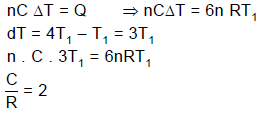JEE Advanced Test- 11 - Question 23

A sample of gas goes from state A to state B in four different manners, as shown by the graphs. Let W be the work done by the gas and DU be change in internal energy along the path AB. Correctly match the graphs with the statements provided.
Column I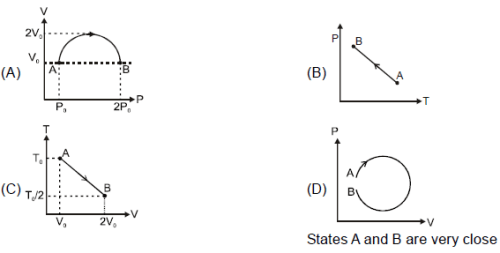Column II

(p) W is positive    (q) ΔU is negative    (r) W is negative    (s) ΔU is positive    (t) heat rejected ΔQ < 0

Detailed Solution for JEE Advanced Test- 11 - Question 23

In (A), V is on vertical axis.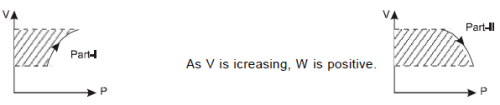As negative work in part-II is greater than positive work in part-I, net work during the process is negative.
Using PV = nRT and as Vremains same for initial and final points of the process, it is obvious that final temp.is greater than initial temperature as pressure has increased. Therefore dU is positive. Hence option (S) is
connected with (A).
Similar arguments can be applied to other graphs.

JEE Advanced Test- 11 - Question 24

A copper rod (initially at room temperature 20°C) of non-uniform cross section is placed between a steam chamber at 100°C and ice-water chamber at 0°C.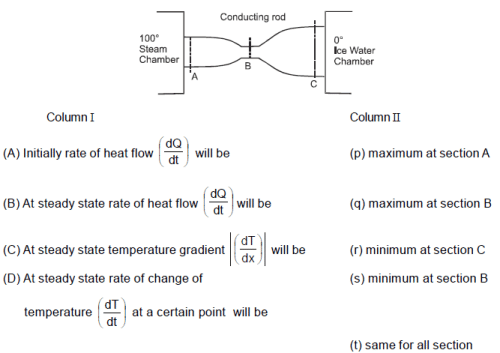Detailed Solution for JEE Advanced Test- 11 - Question 24

(A) Initially more heat will enter through section A but the metal will absorb some heat and less heat will
leave from C.
(B) At steady state heat accumulatron = )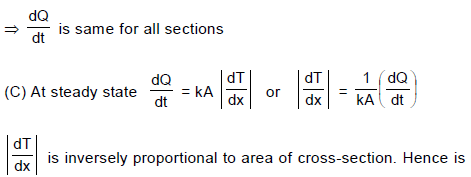maximum at B and minimum at C
(D) At steady state heat accumulation = 0
So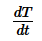=0 for any section.

JEE Advanced Test- 11 - Question 25

Which of the following orders is correct for the size ?

(1) Mg2+ < Na+ < F < Al     (2) Al3+ < Mg2+ < Li+ < K+    (3) Fe4+ < Fe3+ < Fe2+ < Fe    (4) Mg > Al > Si > P

Detailed Solution for JEE Advanced Test- 11 - Question 25

(1) Mg2+, Na+ and Fare isoelectronic and thus follows the order 12Mg2+ < 11Na+ < 9F .
Al belongs to third period and has no charge so it is largest.
Na+ = 102 pm; Mg2+ = 72 pm ; Al = 143 pm, F= 133 pm.
(2) K+ has more number of shells than Mg2+ and AI3+ . AI3+ and Mg2+ are isoelectronic but AI3+ has higher nuclear
charge so AI3+ < Mg2+. Mg2+ and Li+ has diagonal relationship. But due to +2 charge in Mg2+, the Mg2+ is smaller
than Li+. Hence Al3+ is the smallest one. K+ = 1.38 Å, Li2+ = 0.76 Å, Mg2+ = 0.72 Å and Al3+ = 0.535 Å.
(3) As the number of electrons are lost, the attraction between valence shell electrons and nucleus increases. As a
consequence of this the electrons are pulled closer to the nucleus leading to the contraction in size of ions.
(4) Across the period the nuclear charge increases and thus the size of atoms decreases. Mg = 160 pm;
Al = 143 pm; Si = 118 ; P = 110 pm.

JEE Advanced Test- 11 - Question 26

Which reactions involves a change in the electron–pair geometry for the under lined atoms ?

Detailed Solution for JEE Advanced Test- 11 - Question 26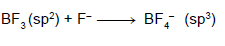JEE Advanced Test- 11 - Question 27

Anhydrous AlCl3 is covalent. From the data given below
Lattice Energy = 5137 KJ/mol.
ΔH hydration for Al3+ = – 4665 KJ/mol
ΔH hydration for Cl = – 381 KJ/mol
Identify the correct statement.

Detailed Solution for JEE Advanced Test- 11 - Question 27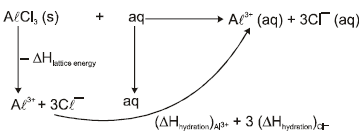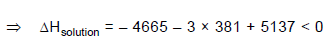Hence AlCl3 will dissolve and solution consists of hydrated Al3+ and Cl ions. In other words higher hydration
energy compensates the higher ionisation energy. As a result, it will exist as hydrated Al3+ and hydrated Cl in aqueous solution.

JEE Advanced Test- 11 - Question 28

In which of the following molecules / ions are all the bond angles not equal ?

Detailed Solution for JEE Advanced Test- 11 - Question 28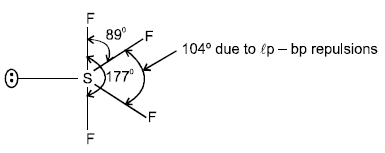Remaining molecules / ions have symmetrical structures ; so have all bonds equal.

JEE Advanced Test- 11 - Question 29

When sodium nitrate is heated at 800ºC :

Detailed Solution for JEE Advanced Test- 11 - Question 29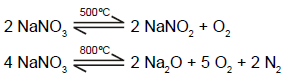JEE Advanced Test- 11 - Question 30

In which of the following  reaction carbon monoxide is obtained as one of the products ?

Detailed Solution for JEE Advanced Test- 11 - Question 30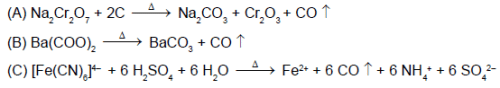JEE Advanced Test- 11 - Question 31

The repeating structural units in silicone is :

Detailed Solution for JEE Advanced Test- 11 - Question 31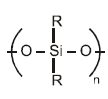JEE Advanced Test- 11 - Question 32

Which of the following product is obtained when boric oxide reacts with hydrogen in presence of Al or Al2Cl6?

Detailed Solution for JEE Advanced Test- 11 - Question 32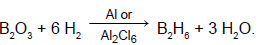*Multiple options can be correct
JEE Advanced Test- 11 - Question 33

Select the correct statement(s).

Detailed Solution for JEE Advanced Test- 11 - Question 33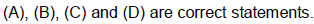*Multiple options can be correct
JEE Advanced Test- 11 - Question 34

Choose the correct options.

Detailed Solution for JEE Advanced Test- 11 - Question 34

(A) Structure is similar to that of ethane. Each N atom is tetrahedrally surrounded by
one N, two H and a lone pair. The two halves of the molecules are rotated 95º about N–N bond and occupy a gauche (non-eclipsed) conformation. The bond length is 1.45 Å.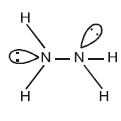(B) Has partial double bond character due to pπ-dπ delocalisation.
(C) OF2=103º (approximate) and OCl2 = 112º (approximate).

(D) Exist in polymeric structure as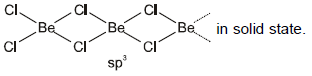*Multiple options can be correct
JEE Advanced Test- 11 - Question 35

In which of the following arrangements, the order(s) is/are according to the property indicated against it?

Detailed Solution for JEE Advanced Test- 11 - Question 35

(A) SbH3 has highest boiling point on account of highest magnitude of vander Waal's force of attraction due to highest molecular mass. Next is of NH3 due to strong H-bonding.
(B) Number of unpaired electron are 5, 4, 3 & 2 respectively.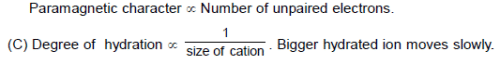D) Bond order is directly proportional to the bond energy.
Bond order of N2 = 3 , N2+ = 2.5 , N22– = 2
But N2 has more electrons in antibonding MO's and thus N2+ is more stable than N2.

*Multiple options can be correct
JEE Advanced Test- 11 - Question 36

Which of the following disproportionate(s) on heating with sodium hydroxide ?

Detailed Solution for JEE Advanced Test- 11 - Question 36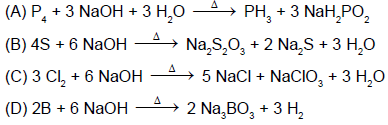JEE Advanced Test- 11 - Question 37

Statement-1 : In general, boiling points rise with increasing molecular weights of the hydrides of 14th group elements because

Statement-2 : The additional mass requires higher temperature for rapid movement of the molecules and the larger number of electrons in the heavier molecules provide larger London forces.

Detailed Solution for JEE Advanced Test- 11 - Question 37

Statement-1 and Statement-2 are True; Statement-2 is a correct explanation for Statement-1.

JEE Advanced Test- 11 - Question 38

Statement-1 : Bond order in a molecule can assume any value positive or negative, integral or fractional when number of electrons in bonding molecular orbitals is greater than that in anti-bonding orbitals.

Statement-2 : Bond order depends on the number of electrons in the bonding and antibonding orbitals.

Detailed Solution for JEE Advanced Test- 11 - Question 38

S-1 : Bond order may be a positve, whole number or fractional values not negative when NB > NA.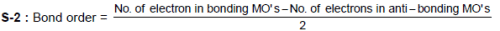JEE Advanced Test- 11 - Question 39

Statement - 1 : Solutions of alkali metals in liquid ammonia are good reducing agents.

Statement - 2 :  They contain free or solvated electrons.

Detailed Solution for JEE Advanced Test- 11 - Question 39

S-1 and S-2 are correct statements and S-2 is correct explanation of S-1.

JEE Advanced Test- 11 - Question 40

Statement - 1 : Grey tin becomes white on cooling to the temperature < 18ºC.

Statement - 2 :  At low temperature grey tin changes to another crystalline form, white tin.

Detailed Solution for JEE Advanced Test- 11 - Question 40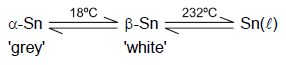JEE Advanced Test- 11 - Question 41

The main group elements i.e. representative elements complete their electron configuration using s and p electrons in the periodic table. These elements range from the most metallic to the most non-metallic, with intermediate properties, the semi-metals,in between. The elements which occur at the two extremes of the periodic table are highly reactive and therefore, these highly reactive elements do not occur in free state ; they usually occur in the combined forms.

Q. Tendency of I–,  Br,  CI and F to be oxidised is in order :

Detailed Solution for JEE Advanced Test- 11 - Question 41

Tendency to lose the electrons increases with the increase in the size of anion. (Valence shell electron(s) to be removed is farther from the nucleus in larger anion).

JEE Advanced Test- 11 - Question 42

The main group elements i.e. representative elements complete their electron configuration using s and p electrons in the periodic table. These elements range from the most metallic to the most non-metallic, with intermediate properties, the semi-metals,in between. The elements which occur at the two extremes of the periodic table are highly reactive and therefore, these highly reactive elements do not occur in free state ; they usually occur in the combined forms.

Q. Select the correct statement (this is with respect to the elements of main group).

Detailed Solution for JEE Advanced Test- 11 - Question 42

(A) Have intermediate values of ionisation energy and electron affinity.
(B) Generally, the elements found in the centre of period for main group elements are metalloids. They generally form amphoteric oxides.
(C) Down the group size of atom increases and electropositive character increases. So metallic character increases.

Across the period, size of atom decreases and electropositive character decreases. So metallic character
decreases and non-metallic character increases.

JEE Advanced Test- 11 - Question 43

The main group elements i.e. representative elements complete their electron configuration using s and p electrons in the periodic table. These elements range from the most metallic to the most non-metallic, with intermediate properties, the semi-metals,in between. The elements which occur at the two extremes of the periodic table are highly reactive and therefore, these highly reactive elements do not occur in free state ; they usually occur in the combined forms.

Q. Beryllium and aluminium exhibit many properties which are similar, But, the two elements differ in :

Detailed Solution for JEE Advanced Test- 11 - Question 43

Beryllium has the maximum vallency +2 while aluminium exhibits its valency as + 3

JEE Advanced Test- 11 - Question 44

Valence shell electron pair repulsion theory (VSEPR) provides a method for predicting the shape of molecules, based on the electron pair electrostatic repulsion. According to VSEPR model, molecules adopt geometries in which their valence electron pairs position themselves as far from each other as possible. The VSEPR model considers double and triple bonds to have slightly greater repulsive effects than single bonds because of the repulsive effect of π  electrons. However, the lone pair creates the maximum repulsive effect.

Q. Which species has the maximum number of lone pairs of electrons on the central atom?

Detailed Solution for JEE Advanced Test- 11 - Question 44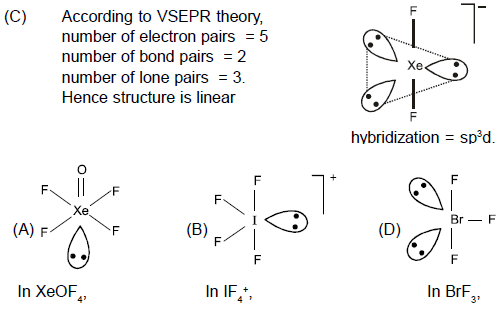JEE Advanced Test- 11 - Question 45

Valence shell electron pair repulsion theory (VSEPR) provides a method for predicting the shape of molecules, based on the electron pair electrostatic repulsion. According to VSEPR model, molecules adopt geometries in which their valence electron pairs position themselves as far from each other as possible. The VSEPR model considers double and triple bonds to have slightly greater repulsive effects than single bonds because of the repulsive effect of π  electrons. However, the lone pair creates the maximum repulsive effect.

Q. Incorrect order about bond angles is :

Detailed Solution for JEE Advanced Test- 11 - Question 45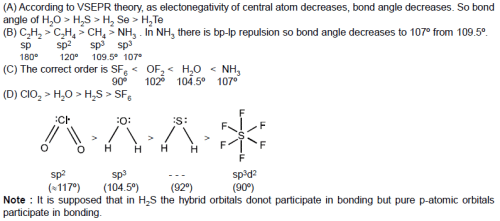JEE Advanced Test- 11 - Question 46

Valence shell electron pair repulsion theory (VSEPR) provides a method for predicting the shape of molecules, based on the electron pair electrostatic repulsion. According to VSEPR model, molecules adopt geometries in which their valence electron pairs position themselves as far from each other as possible. The VSEPR model considers double and triple bonds to have slightly greater repulsive effects than single bonds because of the repulsive effect of π  electrons. However, the lone pair creates the maximum repulsive effect.

Q. Which of the following does not represent the isostructural pair ?

Detailed Solution for JEE Advanced Test- 11 - Question 46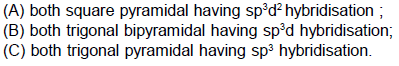JEE Advanced Test- 11 - Question 47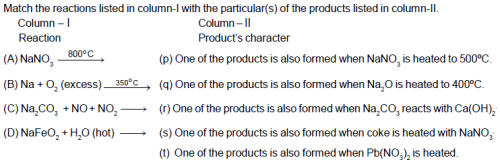Detailed Solution for JEE Advanced Test- 11 - Question 47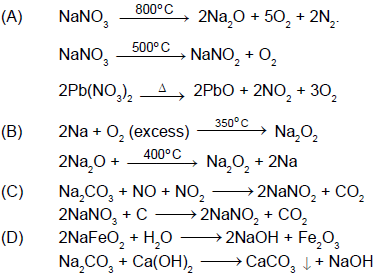JEE Advanced Test- 11 - Question 48

Match the group of species in Column-I with the correctly related characteristic(s) in Column-II.
Column - I                                                           Column - II

(A) CIF5 , BrF4+, IF6                           (p) All molecules/ions are polar in nature.
(B) CIF3 , BrF2+, ICI4–                                  (q) All molecules/ions have same number of lone pair(s) of electrons.
(C) XeF2 , ICI2, I3–                                     (r) All molecules/ions have same oxidation state of central atoms.
(D) CIOF3 , CIF4+, IO2F2                    (s) All molecules/ions have same hybridisation of central atoms.
(t) All molecules/ions have same shape.

Detailed Solution for JEE Advanced Test- 11 - Question 48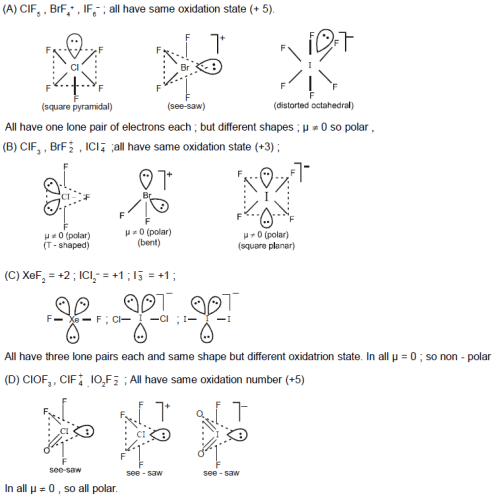JEE Advanced Test- 11 - Question 49

If the pairs of lines x2 + 2xy + ay2 = 0 and ax2 + 2xy + y2 = 0 have exactly one line in common then the joint equation of the other two lines is given by -

Detailed Solution for JEE Advanced Test- 11 - Question 49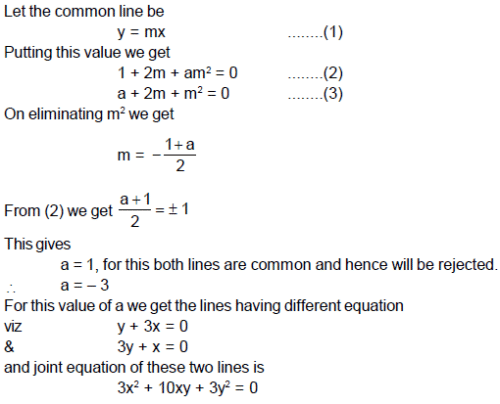JEE Advanced Test- 11 - Question 50

The locus of the point of intersection of the tangents to the circle x = r cos θ, y = r sinθ  at points whose parametric angles differ by π/3, is

Detailed Solution for JEE Advanced Test- 11 - Question 50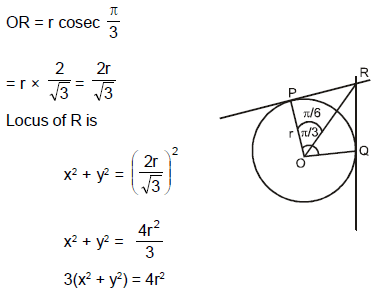JEE Advanced Test- 11 - Question 51

Equation of the circle for which straight lines x2 + 3y + xy + 3x = 0 are normals and size is just sufficient so that it contains the circle x2 + y2 + 2x –  6y + 9 = 0 is -

Detailed Solution for JEE Advanced Test- 11 - Question 51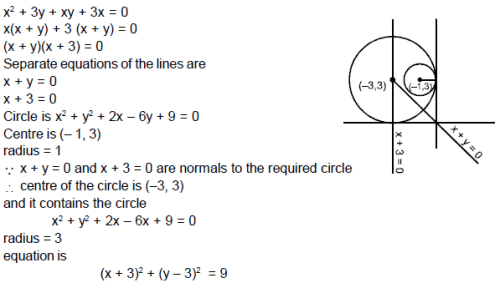JEE Advanced Test- 11 - Question 52

The equation of the incircle of the triangle formed by the axes and the line 4x + 3y = 6 is

JEE Advanced Test- 11 - Question 53

The locus of centres of circles touching the lines x + 2y = 0 and x – 2y = 0 is

JEE Advanced Test- 11 - Question 54

The equation of circumcircle of an equilateral triangle is x2 + y2 + 2gx + 2fy + c = 0 and one vertex of the triangle is (1, 1). The equation of incircle of the triangle is

JEE Advanced Test- 11 - Question 55

The equation of the smallest circle passing through the points of intersection of the line x + y = 1 and the circle x2 + y2 = 9 is

JEE Advanced Test- 11 - Question 56

Equation of straight line ax + by + c= 0, where 3a + 4b + c = 0, which is at maximum distance from (1, –2), is

JEE Advanced Test- 11 - Question 57

If Logx (1/8) = - 3/2, then x is equal to

JEE Advanced Test- 11 - Question 58

The three solutions of the equation f(x) = 0 are - 4, 8, and 11. Therefore, the three solutions of the equation f(2 x) = 0 are

*Multiple options can be correct
JEE Advanced Test- 11 - Question 59

The straight lines u1 = 0, u2 = 0, u3 = 0 and u4 = 0 represent sides of a parallelogram as shown in adjacent figure, then –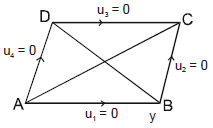Detailed Solution for JEE Advanced Test- 11 - Question 59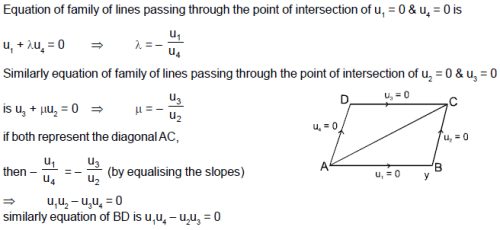JEE Advanced Test- 11 - Question 60

The binary operator ≠ is defined by the following truth table. Which one of the following is true about the binary operator ≠?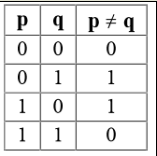JEE Advanced Test- 11 - Question 61

Consider the circular region x2 + y2 = 81, What is the maximum value of the function
f(x, y) = x6 + y2(3x4 + 1) + x2.(3y4 + 1) + y6

Detailed Solution for JEE Advanced Test- 11 - Question 61

Rewrite the function as
f(x, y) = x2 + y2 + (x2 + y2)3
Put x2 + y2 = 81
= 81 + 813.

JEE Advanced Test- 11 - Question 62

Statement - 1 : The point A (3,1,6) is the mirror image of the point B(1,3,4) in the plane x–y+z=5.

Statement - 2 : The plane x – y + z = 5 bisects the line segment joining A(3, 1, 6) and B(1, 3, 4).

Detailed Solution for JEE Advanced Test- 11 - Question 62

Statement-1 is True, Statement-2 is True; Statement-2 is a correct explanation for Statement-1.

JEE Advanced Test- 11 - Question 63

Which one of the following sets of quantum numbers represents the highest energy level in an atom?

JEE Advanced Test- 11 - Question 64

If f (x) = {2x - 3, x ≤ 2} {x, x < 2} then f (1) is equal to

JEE Advanced Test- 11 - Question 65

In the following question, a Statement of Assertion (A) is given followed by a corresponding Reason (R) just below it. Read the Statements carefully and mark the correct answer-
Assertion(A): If M is a n x n matrix with rank n - 1, then M can be made non-singular by changing one element.
Reason(R): An n x n matrix with rank n - 1 has a non-vanishing minor of order n - 1.

JEE Advanced Test- 11 - Question 66

The direction cosine of a vector a=3i+4j+5k in the direction of positive x-axis is

JEE Advanced Test- 11 - Question 67

In the following question, a Statement-1 is given followed by a corresponding Statement-2 just below it. Read the statements carefully and mark the correct answer-
Consider the system of equations
ax+by=0, cx+dy=0, where a, b, c, d ∈ {0,1}
Statement-1:
The probability that the system of equations has a unique solution is 3/2 .
Statement-2:
The probability that the system of equations has a solution is 1.

JEE Advanced Test- 11 - Question 68

If the polar w.r.t.y2 = 4ax touches the ellipse (x22) + (y22)=1, the locus of its pole is

JEE Advanced Test- 11 - Question 69

The A.M., H.M. and G.M. between two numbers are 144/15, 15 and 12, but not necessarily in this order. Then H.M., G.M. and A.M. respectively are

JEE Advanced Test- 11 - Question 70

An explosion breaks a rock into three parts in a horizontal plane. Two of them go off at right angles to each
other. The first part of mass 1 kg moves with a speed of 12 ms–1 and the second part of mass 2 kg moves with 8 ms–1 speed. If the third part files off with 4 ms–1 speed, then its mass is -

JEE Advanced Test- 11 - Question 71

The eccentric angles of the extremities of the latus-rectum intersecting positive x-axis of the ellipse ((x2/a2) + (y2/b2) = 1) are given by

JEE Advanced Test- 11 - Question 72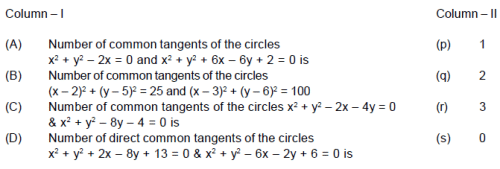Detailed Solution for JEE Advanced Test- 11 - Question 72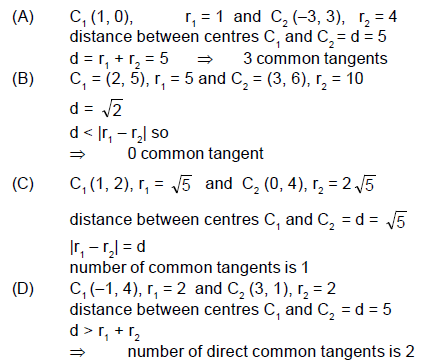## JEE Main & Advanced Mock Test Series

1 videos|354 docs|203 tests(Scan QR code)